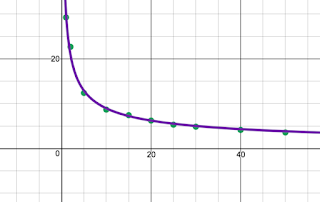## Wednesday, January 24, 2018

### Teach 180: Combining Math Tech (Day 91)

As much as possible, I think it is important for students to discover math for themselves.  Depending on the topic, it can be challenging to think of ways to do that.  Today I combined the technologies of StatKey and Desmos to help students see that the standard error of the sampling distribution of a sample mean is the standard deviation of the population divided by the square root of the sample size.  You know. . . this formula.

First, I assigned students a value for n.  We looked at nine values that between n = 2 to n = 50.  Each student created 400 samples based on their value of n.  The population distribution was the percent of people with internet in various countries. You can see screenshots for the original distribution and for sampling distributions based on sample sizes of n = 4 and n = 16.

On the board each student listed their sample size, mean and standard deviation for their simulated statistic.  It was very easy to see that each simulated distribution was centered at the population mean, and that as the sample size increased, the variability decreased.  Was there a formula for calculating the variability or standard deviation from the sample size?  To see what the relationship might be, we plotted the following in desmos and did a regression.As a class we noticed that a is close to the standard deviation of the population, 29.259, and b is about 0.5.  This also allowed us to review regression and the fact that this equation is based on sample data from our simulations.  If we ran the simulations again, we would get slightly different data and as a result, a slightly different equation.  One thing to make this lesson better would be to have students discover this for themselves individually or in groups.  However, crowdsourcing this discovery as a class worked fairly well.